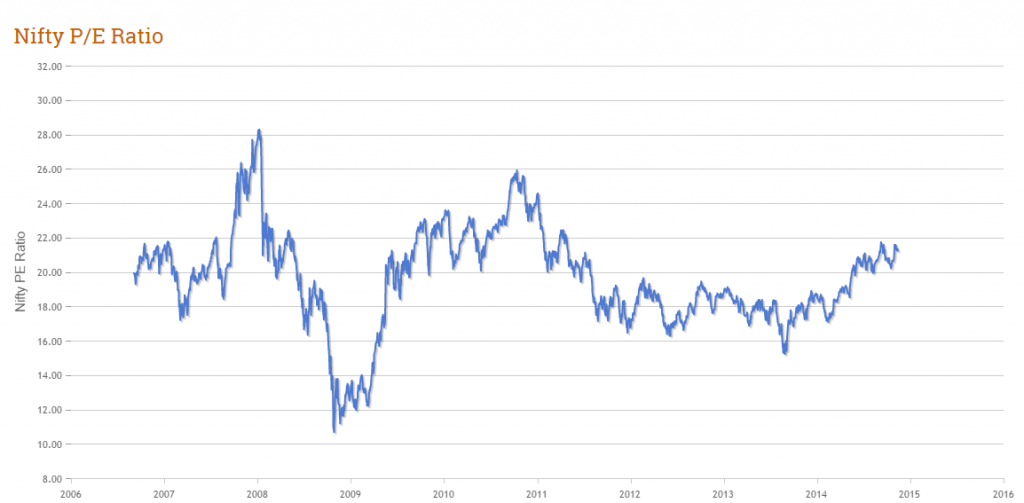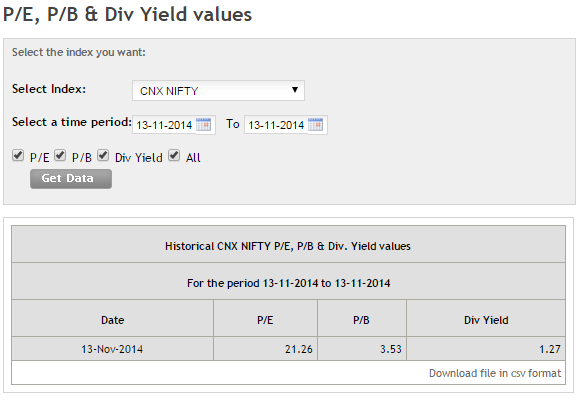# The Study of Stock Market through Fundamental Analysis

## 11.1 - Overview of Valuation Ratio

In general, valuation is the estimation of the 'worthiness' of something. When discussing investments, "something" refers to the stock's price. The business's value is what really matters when making investment decisions. The price you pay for a business to buy determines its value. A mediocre business that has a low valuation can be a great option to invest in, rather than an exciting business that has a high valuation.

We can use valuation ratios to get a feel for how market participants value stock prices. These ratios can help investors understand the value of the stock price. Valuation ratios are used to compare the stock's price with the benefits it offers. As with all other ratios, the valuation ratios of a company should be evaluated along with its competitors.

Value ratios are often calculated as a ratio between the share price of a company and a particular aspect of its financial performance. The following three valuation ratios are important:

1. Ratio Price to Sales
2. Ratio Price to Book Value (P/BV).
3. Price to Earnings Ratio (P/E).

Let's continue with the Amara Raja Batteries Limited, (ARBL), example. We will use these ratios to determine how ARBL does. It is important to know the stock price of ARBL in order to calculate the valuation ratios. ARBL is currently trading at Rs.661 per Share as I write this chapter about 28 th Oct 2014.

To calculate the ratios above, we also need to know the ARBL total shares outstanding. The same formula was used in chapter 6 to calculate the above ratios. The total number outstanding shares is 17,08,12,500.

Ratio Price to Sales

Investors may choose to use earnings rather than sales in many cases when valuing their investments. Some companies may be experiencing a cyclical low during their earnings cycle, so the earnings figure might not be accurate. Due to accounting rules, it is possible for a profitable company to appear to have no earnings due to the large write-offs that are applicable to this industry. Investors would rather use this ratio. This ratio is calculated by comparing the stock price of the company and the company's average sales per share. This formula calculates the P/S ratio:

Price to Sales Ratio =

Current Share Price divided by Sales per Share

Sales per share =

Total Revenues divided by Total number of shares

ARBL's P&L statement reveals this:

Total Revenue = Rs.3482 Crore

17.081 Cr = Number of shares

Sales per share = 3482/ 17.081

The Sales per Share = Rs. 203.86

ARBL makes Rs.203.86 for each share that is outstanding.

Price to Sales Ratio = 661/203.86

= 3.24x, 3.24x or 3.24x

A P/S ratio 3.24 times means that for every Rs.1 in sales, the stock's value is Rs.3.24 more. The firm's valuation will rise if the P/S ratio is higher. To get an accurate idea of the stock's price, one must compare its P/S ratio to those of other companies.

This is what you should remember when calculating the P/S Ratio. Suppose there are 2 companies selling the same product. Each company generates Rs.1000 in revenue. Company A keeps Rs.250 for PAT, while Company B keeps Rs.150. This means that Company A's profit margin is 25% while Company B's profit margin is 15%. Therefore, Company A's sales is more valuable than that of Company B. Therefore, Company A may trade at a higher price per share. This valuation could be justified as Company A makes more sales than it loses.

If you believe a company is trading at an elevated valuation, make sure to verify the profit margin.

Ratio Price to Book Value (P/BV).

Before we can understand the Price to book Value ratio, it is important to first understand what the term "Book Value" means.

Imagine a company that must close its doors and liquidate all of its assets. What is the minimum value that the company will receive upon liquidation? This is the "Book Value" for the company.

The book value of a company is simply the money that remains after the company has paid its obligations. The book value is the company's salvage value. Let's say the book value for a company is Rs.200Crs. This is the money that the company can expect after it sells all its assets and settles all its debts. The book value is usually expressed per share. If the book value per share was Rs.60, then Rs.60 per shared is what the shareholder should expect in the event of liquidation. You can calculate the 'Book Value (BV) as follows:

BV = [Share capital + Reserves (excluding the revaluation reserves)/ Total number of shares

Let's do the same with ARBL.

We know this from ARBL's balance sheets:

Share Capital = Rs.17.1 Crs

Reserves = Rs.1345.6 Crore

Reserves for Revaluation = 0

Number of shares: 17.081

The Book Value per Share = [17.1+1345.6-0] / 17.081

Shares: Rs.79.8

The shareholders can therefore expect Rs.79.8 per share if ARBL liquidates all its assets and pays off its debt.

If we divide the stock price at the moment by the book price per share, then we get the price at the firm's books value. The ratio P/BV shows how often the stock trades above and below the firm's value. The stock will be more expensive if the ratio is higher.

Let's calculate ARBL. We know this:

ARBL's stock price is Rs.661 per share

ARBL BV = 79.8/share

P/BV = 661/79.8

8.3x, 8.3x

ARBL trades at 8.3 times its book price.

A high ratio could mean that the firm's equity/book value is undervalued. Low ratios could indicate that the company is undervalued in relation to its equity/book value.

Price to Earnings (P/E) Ratio

Perhaps the most well-known financial ratio is the Price to Earnings ratio. Everyone loves to see the stock's P/E ratio. The P/E ratio is often referred to as the "financial ratio star" because of its popularity.

Divide the P/E of a stock byCurrent stock priceBy theEarnings per Share(EPS). Before we get into the PE ratio, let's first understand what "Earnings Per Share" (EPS), stands for.

EPS is a measure of a company's profitability per share. Let's say that a company with 1000 shares outstanding makes a profit of Rs.200000/. If you calculate the earnings per share, it would be:

=200000 / 1000

Shares: Rs.200

The EPS is a measure of profits per share. It is obvious that a higher EPS means a better return for shareholders.

Divide the current market price by EPS to get the Price-to-Earnings ratio. Measurement of willingness of the market participants to pay for the stock for every rupee that companies earn is the P/E ratio. If a firm's P/E is 15 it means that for every unit of profit earned, the market participants will pay 15 times. Stocks that have a higher P/E are more expensive.

Let's calculate the P/E of ARBL. From its annual report, we know -

PAT = Rs.367Crs

Total number of shares = 17.081 Cr

EPS = Total Number of Shares

= 367 / 17.081

= Rs.21.49

ARBL's current market price = 661

Therefore, P/E = 661/21.49

= 30.76

ARBL generates a profit of Rs.30.76 per unit. Market participants will pay Rs.30.76 for a share.

Let's say ARBL's prices jump to Rs.750, while the EPS stays at Rs.21.49. The new P/E would then be:

= 750/21.49

= 34.9 Times

The EPS remained flat at Rs.21.49 per shares, but the stock's P/E jumped. What do you think?

Evidently, the P/E Ratio rose due to the rise in stock prices. We know that stock prices increase when there are higher expectations.

Remember that the P/E Ratio uses 'earnings" as its numerator. These are the key points to remember when calculating the P/E Ratio:

1. The stock's price to earnings (P/E) indicates its value. Avoid stocks with high valuations. I don't like stocks trading at higher valuations than 25 to 30 times their earnings. This is regardless of whether the stock is from a company or a sector.
2. The 'Earnings denominator' in the P/E ratio can be modified.
3. You should ensure that the company does not change its accounting policy often. This is one of the ways the company attempts to manipulate its earnings.
4. Pay attention to how depreciation will be treated. Allowing for less depreciation can increase earnings.
5. It is clear that something is wrong if the company's earnings increase but not its cash flow and sales.

## 11.2 - The Index Valuation

Stock market indices like the CNX Nifty 50 and the BSE Sensex have their valuations calculated by the Dividend Yield, P/E and P/B ratios. The index valuation is usually published by the stock exchanges daily. We can get a feel for how expensive or cheap the market is trading. The CNX 50 P/E ratio is calculated by the National Stock Exchange. This calculates the total market capitalization of all 50 stocks, and then divides this amount by the total earnings for all 50 stocks. The Index P/E ratio is a measure of market state as it is perceived by market participants. The historical chart for Nifty 50 P/E is shown below.6. We can draw some important conclusions from the P/E chart.

7. The peak Index valuation was 28x in early 2008, and what followed was a major crash on the Indian markets
8. The corrections brought the valuation down to 11x (late 2008/early 2009). This was the lowest Indian market valuation in recent years
9. The average ratio of P/E for Indian Indices is 16x to 20x.
10. Today (2014), we trade at 22x. This is higher than the average P/E ratio
11. These observations lead to the following conclusion:

12. When the P/E valuations of stocks are higher than 22x, one must be careful when investing in stocks
13. The best time to invest is historically when market valuations are below 16x.
14. The NSE website allows you to easily check the Index P/E value each day.

Click on Products > Indices > Historic Data to go to NSE's homepage.

Enter today's date in the search field to get the most recent P/E valuation for the market. This information is updated by the NSE every day at 6:00 PM.

Here's a quick snapshot of the search results -As of today (13 th Nov 2014), India's market trades close to the higher end P/E range. History suggests that investors should be cautious at this level.

## To Summarize

15. In general, valuation is the estimation of the 'worthiness' of something.
16. The inputs to calculate valuation ratios are the P&L statement as well as the Balance Sheet.
17. The Price to sales ratio is a comparison of the stock price and the company's average earnings per share.
• Simply put, sales per share equals the number of shares divided by the sales.
18. A company that has a higher profit margin is more profitable than a company that has a lower profit margin.
19. The 'Book Value' is the money that remains after a company has paid its obligations.
20. Book value is often expressed per share.
21. The Price/BV indicator indicates how often the stock price trades above and beyond the firm's books value.
22. EPS is a measure of profitability on a per share basis.
23. The P/E ratio is a measure of the willingness of market participants to buy stock. It helps keep company earnings in perspective.
24. When analyzing the P/E ratio, one must be careful about earning manipulation.
25. The valuation of the Indices can be determined by the Dividend Yield, P/E or P/B ratio.
26. When the Index trades at 22x or more, it is prudent to be cautious.
27. When the index trades at 16x or less, a valuation is attractive.
28. NSE publishes daily index valuations on its website